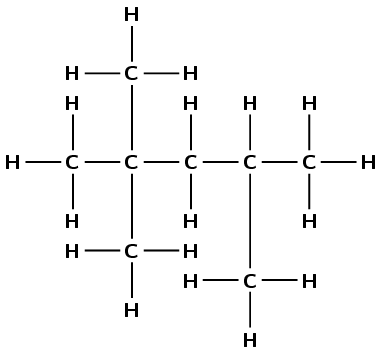Home Practice
For learners and parents For teachers and schools
Textbooks
Full catalogue
Pricing SupportLog in

We think you are located in United States. Is this correct?

# End of chapter exercises

Textbook Exercise 4.33

Give one word or phrase for each of the following descriptions:

The name of the homologous series to which 2-methylpropene belongs.

Alkenes

The name of the functional group that gives alcohols their properties.

Hydroxyl group

The group of organic compounds that have acidic properties.

Carboxylic acid

The IUPAC name of the organic compound that is found in vinegar.

Ethanoic acid

The name of the organic compound that is found in alcoholic beverages.

Ethanol

When 1-propanol is oxidised by acidified potassium permanganate, the possible product formed is:

• propane

• propanoic acid

• methyl propanol

• propyl methanoate

(IEB 2004)

propanoic acid

What is the IUPAC name for the compound represented by the following structure?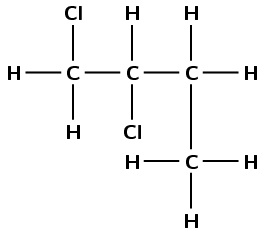• 1,2-dichlorobutane

• 2,2-dichlorobutane

• 1,2-dichloro-3-methylpropane

• 1,1-dichloro-3-methylpropane

(IEB 2003)

1,2-dichlorobutane

Give the structural representation and IUPAC name of all possible isomers for $$\text{C}_{6}\text{H}_{14}$$ (hint: there are 5)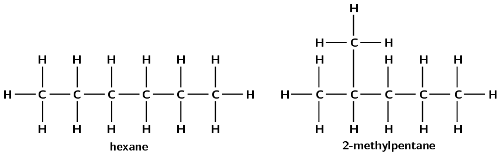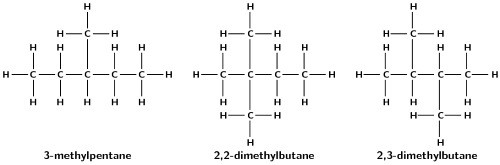Write balanced equations for the following reactions:

Ethene reacts with bromine (use condensed structural representations)

$$\text{CH}_{2}\text{CH}_{2} + \text{Br}_{2}$$ $$\to$$ $$\text{CH}_{2}(\text{Br})\text{CH}_{2}(\text{Br})$$

ethene + bromine $$\to$$ 1,2-dibromoethane

The complete combustion of ethyne gas (used molecular formula)

$$2\text{C}_{2}\text{H}_{2}(\text{g}) + 5\text{O}_{2}(\text{g})$$ $$\to$$ $$4\text{CO}_{2}(\text{g}) + 2\text{H}_{2}\text{O}(\text{g})$$

Ethanoic acid ionises in water

$$\text{CH}_{3}\text{COOH}(\text{ℓ}) + \text{H}_{2}\text{O}(\text{ℓ})$$ $$\to$$ $$\text{CH}_{3}\text{COO}^{-}(\text{aq}) + \text{H}_{3}\text{O}^{+}(\text{aq})$$

The table below gives the boiling point of ten organic compounds.

 Compound Molecular formula Boiling Point (°C) $$\text{1}$$ methane $$\text{CH}_{4}$$ $$-\text{162}$$ $$\text{2}$$ ethane $$\text{C}_{2}\text{H}_{6}$$ $$-\text{89}$$ $$\text{3}$$ propane $$\text{C}_{3}\text{H}_{8}$$ $$-\text{42}$$ $$\text{4}$$ butane $$\text{C}_{4}\text{H}_{10}$$ $$\text{0}$$ $$\text{5}$$ pentane $$\text{C}_{5}\text{H}_{12}$$ $$\text{36}$$ $$\text{6}$$ methanol $$\text{CH}_{4}\text{O}$$ $$\text{64,7}$$ $$\text{7}$$ ethanol $$\text{C}_{2}\text{H}_{6}\text{O}$$ $$\text{78,4}$$ $$\text{8}$$ propan-1-ol $$\text{C}_{3}\text{H}_{8}\text{O}$$ $$\text{97}$$ $$\text{9}$$ propan-1,2-diol $$\text{C}_{3}\text{H}_{8}\text{O}_{2}$$ $$\text{188}$$ $$\text{10}$$ propan-1,2,3-triol $$\text{C}_{3}\text{H}_{8}\text{O}_{3}$$ $$\text{290}$$

The following questions refer to the compounds shown in the above table.

To which homologous series do the following compounds belong?

Compounds 1 - 5

Alkanes

Compounds 6 - 10

Alcohols

Which of the above compounds are gases at room temperature?

Methane, ethane, propane and butane (i.e. compounds 1 - 4)

What is the reason for the trend of increasing boiling points seen in compounds 1 to 5?

As the alkanes increase in chain length so does their surface area. This leads to stronger intermolecular forces and an increase in boiling point.

Despite the fact that the length of the carbon chain in compounds 8,9 and 10 is the same, the boiling point of propan-1,2,3-triol is much higher than the boiling point of propan-1-ol. What is responsible for this large difference in boiling point?

As each hydroxyl group is added the strength of the intermolecular forces increases. The compound can form more hydrogen bonds. This leads to an increase in the boiling point.

Give the IUPAC name and the structural representation of an isomer of butane.

2-methylpropane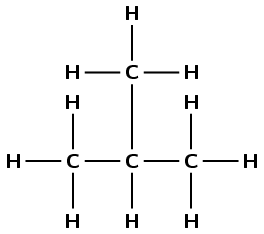Which one of the compounds is used as a reactant in the preparation of the ester ethyl methanoate?

Ethanol (7)

Using structural representation, write an equation for the reaction which produces ethyl methanoate.

(IEB 2004)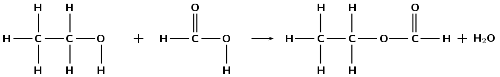Refer to the numbered diagrams below and then answer the questions that follow.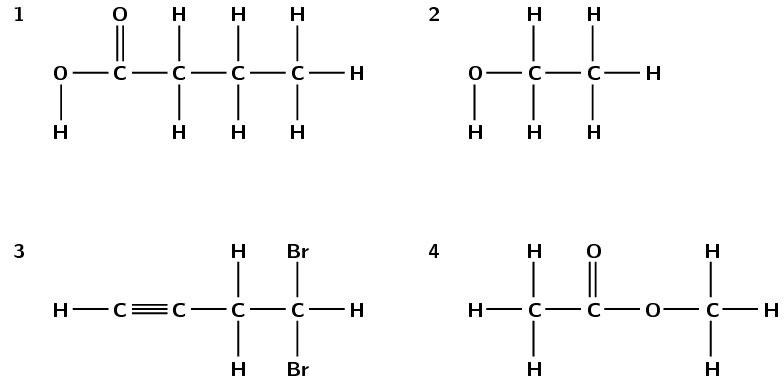Which one of the above compounds is produced from the fermentation of starches and sugars in plant matter?

compound 2, ethanol

To which one of the following homologous series does compound 1 belong?

1. esters

2. alcohols

3. aldehydes

4. carboxylic acids

iv) carboxylic acids

The correct IUPAC name for compound 3 is...

1. 1,1-dibromobut-3-yne

2. 4,4-dibromobut-1-yne

3. 2,4-dibromobut-1-yne

4. 4,4-dibromoprop-1-yne

ii) 4,4-dibromobut-1-yne

What is the correct IUPAC name for compound 4?

1. propanoic acid

2. ethyl methanoate

3. methyl ethanoate

4. methyl propanoate

IEB 2005

iii) methyl ethanoate

Explain the term homologous series.

A homologous series is a series of compounds with the same general formula. All molecules in this series will contain the same functional groups. For example the alkanes are a homologous series: they have the general formula $$\text{C}_{\text{n}}\text{H}_{2\text{n}+2}$$; for every extra carbon atom there are two extra hydrogen atoms.

A mixture of ethanoic acid and methanol is warmed in the presence of concentrated sulfuric acid.

Using structural representations, give an equation for the reaction which takes place.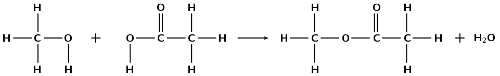What is the IUPAC name of the organic compound formed in this reaction?

methyl ethanoate

Consider the following hydrocarbon: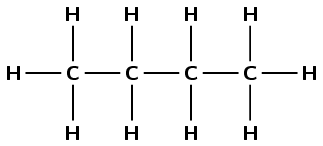Give the IUPAC name for this compound.

There are four carbon atoms, therefore the prefix is but-. There are only single carbon-carbon bonds and no other functional group, therefore this is an alkane and the suffix is -ane.

butane

Give the balanced equation for the complete combustion of this compound in excess oxygen (use molecular formulae).

(IEB Paper 2, 2003)

Write the unbalanced equation:

$$\text{C}_{4}\text{H}_{10}(\text{g}) + \text{O}_{2}(\text{g})$$ $$\to$$ $$\text{CO}_{2}(\text{g}) + \text{H}_{2}\text{O}(\text{g})$$

Balance the equation:

$$2\text{C}_{4}\text{H}_{10}(\text{g}) + 13\text{O}_{2}(\text{g})$$ $$\to$$ $$8\text{CO}_{2}(\text{g}) + 10\text{H}_{2}\text{O}(\text{g})$$

Consider the organic compounds labelled A to D.

A. $$\text{CH}_{3}\text{CH}_{2}\text{CH}_{2}\text{CH}_{2}\text{CH}_{2}\text{CH}_{3}$$

B. $$\text{C}_{6}\text{H}_{6}$$

C. $$\text{CH}_{3}-\text{Cl}$$

D.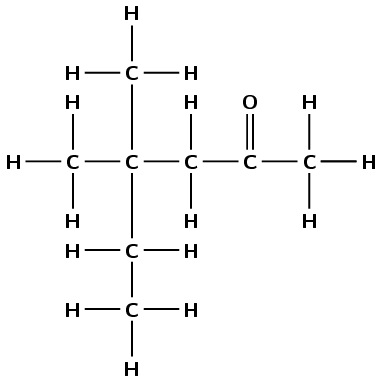Write a balanced chemical equation for the preparation of compound C using an alkane as one of the reactants.

$$\text{CH}_{4} + \text{Cl}_{2} + \text{light}$$ $$\to$$ $$\text{CH}_{3}\text{Cl} + \text{HCl}$$

Write down the IUPAC name for compound D.

There are six carbon atoms in the longest chain containing the functional group so the prefix is hex-. The compound is a ketone and the ketone carbon is the second carbon atom, so the suffix is -2-one. There are 2 methyl groups attached to the fourth carbon atom (4,4-dimethyl). The compound is 4,4-dimethylhexan-2-one.

Write down the structural representation of an isomer of compound A that has only FOUR carbon atoms in the longest chain.

The molecular formula of compound A is $$\text{C}_{6}\text{H}_{14}$$.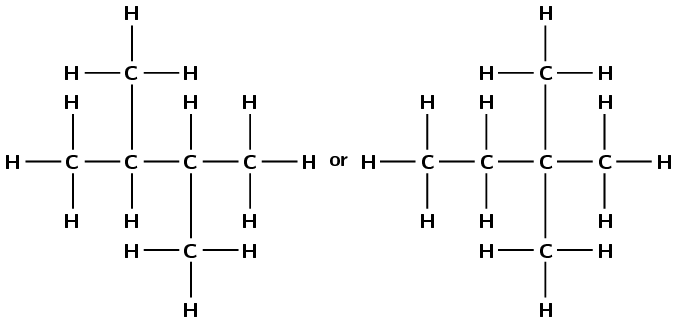Note that these are the only two possibilities.

Write down the structural representation of compound B.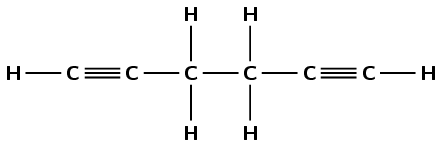or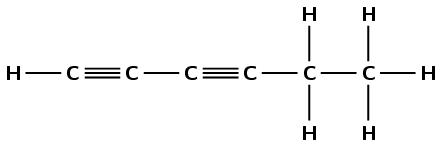or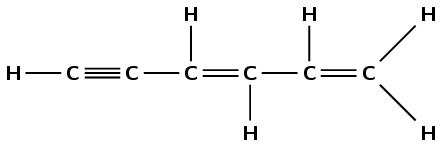Any one of the above structures is reasonable. Learners can also draw benzene.

Chlorine and ethane react to form chloroethane and hydrochloric acid.

Write a balanced chemical equation for this reaction, using molecular formulae.

$$\text{Cl}_{2}(\text{g}) + \text{C}_{2}\text{H}_{6}(\text{g}) + \text{light}$$ $$\to$$ $$\text{C}_{2}\text{H}_{5}\text{Cl}(\text{g}) + \text{HCl}(\text{ℓ})$$

Give the structural representation of chloroethane.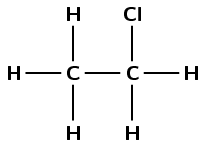What type of reaction has taken place in this example?

Substitution

Petrol is in fact not pure $$\text{C}_{8}\text{H}_{18}$$ but a mixture of various alkanes. The octane rating of petrol refers to the percentage of the petrol which is $$\text{C}_{8}\text{H}_{18}$$. For example, $$\text{93}$$ octane fuel contains 93% $$\text{C}_{8}\text{H}_{18}$$ and 7% other alkanes. The isomer of $$\text{C}_{8}\text{H}_{18}$$ referred to in the octane rating is in fact not octane but 2,2,4-trimethylpentane.

Write a balanced equation for the chemical reaction which takes place when $$\text{C}_{8}\text{H}_{18}$$ burns in excess oxygen (complete combustion).

$$2\text{C}_{8}\text{H}_{18}(\text{ℓ}) + 25\text{O}_{2}(\text{g})$$ $$\to$$ $$16\text{CO}_{2}(\text{g}) + 18\text{H}_{2}\text{O}(\text{g}) + \text{heat}$$

Give the general formula of the alkanes.

$${\text{C}}_{n}{\text{H}}_{2n+2}$$

Define the term isomer.

Isomers are two (or more) organic compounds that have the same molecular formula but a different structural formula.

Use the information given in this question and your knowledge of naming organic compounds to deduce and draw the full structural formula for 2,2,4-trimethylpentane.(I.E.B.)